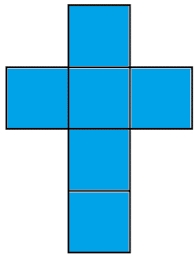# Cube surface and volume

Find the surface of the cube with a volume of 27 dm3.

S =  54 dm2

### Step-by-step explanation:Did you find an error or inaccuracy? Feel free to write us. Thank you!Tips to related online calculators
Tip: Our volume units converter will help you with the conversion of volume units.

## Related math problems and questions:

• Cube V2SThe volume of the cube is 27 dm cubic. Calculate the surface of the cube.
• Area to volumeIf the surface area of a cube is 486, find its volume.
• The cubeThe cube has a surface area of 216 dm2. Calculate: a) the content of one wall, b) edge length, c) cube volume.
• Area of a cubeCalculate the surface area of a cube if its volume is equal to 729 cubic meters.
• Cube surfce2volumeCalculate the volume of the cube if its surface is 150 cm2.
• The cubeThe cube has a surface area of 486 m ^ 2. Calculate its volume.
• Cube surface and volumeThe surface of the cube is 500 cm2, how much cm3 will be its volume?
• The cubeThe cube has a surface of 600 cm2. What is its volume?
• The volume 2The volume of a cube is 27 cubic meters. Find the height of the cube.
• Length of the edgeFind the length of the edge of a cube with a cm2 surface and a volume in cm3 expressed by the same number.
• Find theFind the surface area of a regular quadrilateral pyramid which has a volume of 24 dm3 and a height of 45 cm.
• Volume and areaWhat is the volume of a cube which has an area of 361 cm2?
• Rectangular cuboidThe rectangular cuboid has a surface area 5334 cm2, and its dimensions are in the ratio 2:4:5. Find the volume of this rectangular cuboid.
• Cube 6Volume of the cube is 216 cm3, calculate its surface area.
• Two rectangular boxesTwo rectangular boxes with dimensions of 5 cm, 8 cm, 10 cm, and 5 cm, 12 cm, 1 dm are to be replaced by a single cube box of the same cubic volume. Calculate its surface.
• Prism XThe prism with the edges of the lengths x cm, 2x cm, and 3x cm has volume 20250 cm3. What is the area of the surface of the prism?
• TerezaThe cube has an area of base 256 mm2. Calculate the edge length, volume, and area of its surface.